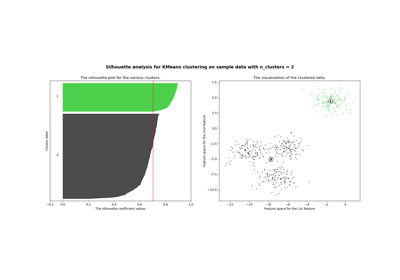# sklearn.metrics.silhouette_samples¶

sklearn.metrics.silhouette_samples(X, labels, *, metric='euclidean', **kwds)[source]

Compute the Silhouette Coefficient for each sample.

The Silhouette Coefficient is a measure of how well samples are clustered with samples that are similar to themselves. Clustering models with a high Silhouette Coefficient are said to be dense, where samples in the same cluster are similar to each other, and well separated, where samples in different clusters are not very similar to each other.

The Silhouette Coefficient is calculated using the mean intra-cluster distance (a) and the mean nearest-cluster distance (b) for each sample. The Silhouette Coefficient for a sample is (b - a) / max(a, b). Note that Silhouette Coefficient is only defined if number of labels is 2 <= n_labels <= n_samples - 1.

This function returns the Silhouette Coefficient for each sample.

The best value is 1 and the worst value is -1. Values near 0 indicate overlapping clusters.

Read more in the User Guide.

Parameters
Xarray-like of shape (n_samples_a, n_samples_a) if metric == “precomputed” or (n_samples_a, n_features) otherwise

An array of pairwise distances between samples, or a feature array.

labelsarray-like of shape (n_samples,)

Label values for each sample.

metricstr or callable, default=’euclidean’

The metric to use when calculating distance between instances in a feature array. If metric is a string, it must be one of the options allowed by sklearn.metrics.pairwise.pairwise_distances. If X is the distance array itself, use “precomputed” as the metric. Precomputed distance matrices must have 0 along the diagonal.

**kwdsoptional keyword parameters

Any further parameters are passed directly to the distance function. If using a scipy.spatial.distance metric, the parameters are still metric dependent. See the scipy docs for usage examples.

Returns
silhouettearray-like of shape (n_samples,)

Silhouette Coefficients for each sample.

References

1

Peter J. Rousseeuw (1987). “Silhouettes: a Graphical Aid to the Interpretation and Validation of Cluster Analysis”. Computational and Applied Mathematics 20: 53-65.

2

Wikipedia entry on the Silhouette Coefficient

## Examples using sklearn.metrics.silhouette_samples¶Selecting the number of clusters with silhouette analysis on KMeans clustering¶